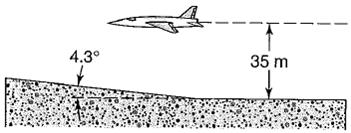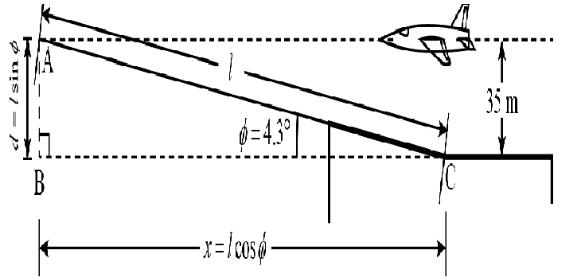Courses

# Notes | EduRev

## JEE : Notes | EduRev

The document Notes | EduRev is a part of the JEE Course Mock Test Series for JEE Main & Advanced 2021.
All you need of JEE at this link: JEE

Question 1: A certain computer hard disk drive is rated to withstand an acceleration of 100g without damage. Assuming the drive decelerates through a distance of 2 mm when it hits the ground, from how high can you drop the drive without running it?
Concept:
The height say y from which the disk falls freely, can be related to its final speed before collision say vy and the initial speed v0y as:
(vy)– (v0y)2 = 2gy
From this expression, we calculate the speed of the disk just before it hits the ground but in terms of height y.
If you assume that the initial speed of the disk before it hits the ground is v'0y, the final speed to be v'y and the distance travelled during the collision is given by y', then the acceleration of the disk during collision is given using the relation: (v'y)2 – (v'0y)2 = 2ay'
Since the speed of the disk before collision is known from above, we can equate the limiting value of acceleration to account for the height y from which the disk can be thrown without damaging it.
Solution:
From the equation of kinematics, we have
y = v0yt + ½ at2 (for y= 0)
vy= v0y + at
Where vy represents the final speed of the disk, a is its acceleration, v0y is the initial speed and  is the time taken by the disk to attain the final speed vy.
From the second equation, the time t is given as: t = (vy- v0y) /a
Substitute the value in first equation
y = v0y [(v- v0y)/a] + ½ a [(v- v0y)/a]2
= [(v- v0y)/a] [v0y + ½ a[(v- v0y)/a]]
= [(v- v0y)/a] [(2 v0y+ v- v0y)/2]
= [(v- v0y)/a] [(vy+v0y)/2]
So,
2ay = (v- v0y)(v+ v0y)
= (vy)2 – (v0y)2
Since the disk accelerates under the action of gravity and we take the convention of downward motion to be positive, we can equate a = g in the equation above as:
(vy)2 – (v0y)2 = 2gy      …… (1)
Substitute the given value of v0y to be zero, the above equation can be written as:
(vy)2 – (0 m/s)2 = 2gy
(vy)2 = 2gy     …… (2)
Assume that the distance travelled by the disk during collision is y', the speed of the disk just before the contact is v'0y, and the final speed after the collision is v'y, such that the disk experience an acceleration a, then equation (1) can be written as:
(v'y)2 – (v'0y)2 = 2ay'
Since the disk will come to rest after colliding, hits final speed v'y is zero. Also the initial speed before collision is equal to vy calculated above.
Given that the disk crushes to a depth of  2 mm, the height y' can be equated to it.
Substitute the appropriate values in the equation above,
(0 m/s)– (vy)2 = 2a (2 mm)
Substitute the value of  (vy)2  from equation (2),
-2gy = 2a (2mm)
Given that the limit to the deceleration that the disk can withstand during the collision is 100 g,  we can equate a = 100 g as,
-2gy = 2(-100 g)(2mm)
y = 100 (2 mm) (10-3 m/1 mm)
So, y = 0.2 m
Therefore if the disk is thrown from height 0.2 m the disk will start to damage, therefore the person can throw the disk from any height which is less than 0.2 m and avoid damaging it.

Question 2: A high-performance jet plane, practicing radar avoidance maneuvers, is in horizontal flight 35 m above the level ground. Suddenly, the plane encounters terrain that slopes gently upward at 4.3°, an amount difficult to detect; see below figure-1. How much time does the pilot have to make a correction if the plane is to avoid flying into the ground? The airspeed is 1300 km/h.Solution:-
Given:
Inclination of slope, ? = 4.3°.
Altitude of plane,  h = 35 m.
Speed of air, vair = 1300 km/h.
Let us assume that the length of slope to the point where the plane meets the slope is given by length l. Also, the speed of the plane is equal in magnitude with that of the speed of air, therefore the speed of the plane (say vplane) is equal to 1300 km/h.
The figure below shows the motion of the plane and various other variablesFrom the figure one can see that the altitude (say d) of the slope is given in terms of length l of slope and the angle ? as:
d = l sin ?
If the plane were to collide with the slope then the following condition should be fulfilled:
h - d = 0
d = h
Substitute the value of d and h to have,
l sin ? = 35 m
l = 35 ml sin (4.3°)
So, l = 466.7 m
Therefore the length of the slope after which the plane collides is 466.7 m.
Now, to calculate the horizontal distance (say x) that the plane will travel if it collides with the slope, we calculate the base of triangle ABC as
From triangle ABC, we have
x = l cos ?
Substitute the value of l and ?, to have
x = (466.7 m) [cos (4.3°)]
= 465.3 m
Therefore the horizontal distance at which the plane will collide with the slope is 465.3 m.
The time (say t) that the plane had before it run into the inclined ground ahead is given as:
t = x/vplane
Substituting the value of x  and vplane in the equation t = x/vplane, we get,
t = (465.3 m)/(1300 km/h)
= (465.3 m)/[(1300 km/h) (103 m/1 km) (1 h/3600 s)]
=(465.3 m)/(361.1 m/s)
= 1.28 s
Rounding off to two significant figures,
t = 1.3 s
Therefore the plane has 1.3 s before it will run into the ground, and avoid the collision.

Question 3:-A juggler juggles 5 balls with two hands. Each ball rises 2 meters above her hands. Approximately how many times per minute does each hand toss a ball?
Concept:
First calculate the time for which a ball stays in air. Suppose that the time taken by the ball to reach the juggler hand from height say y is t. Since the balls begin with initial speed say v0y of zero, the time t  can be calculated as:
y = v0yt + ½ gt2
y = ½ gt    (for v0y = 0 m/s)        …… (1)
The convention chosen is such that the downward motion is consider as positive.
The time (t) calculated above accounts for the time taken by the ball to reach the hand of the juggler from the maximum height it attains, therefore to calculate the time say t', for which the ball stays in the air, multiple the time (t) by 2.
By the time the ball left from one hand and reached the other, the juggler must have thrown 5 balls so that he can be ready to throw the ball reaching his hand again.Therefore, if the juggler has thrown 5 balls in time t', he would throw (5/t') (1 min) times each ball with his two hands in 1 min.
Thus the number of times say n, the ball is tossed by each hand is given as:
n = (5/t')(1 min)/2         …… (2)
Solution:
Given that the ball rise 2 m above the hand, the distance y travelled by the ball when he descends from the maximum height to reach the juggler hand is 2 m. Also the free fall acceleration is 9.81 m/s2.
Substitute the given values in equation (1),
y = ½ gt2
2 m = ½ (9.81 m/s2) t2
t = √4 m/(9.81 m/s2)
= 0.63 s
The time (t') for which a ball stays in the air is given by multiplying t by 2 as:
t ' = 2t
= 2 (0.63 s)
= 1.27 s
The juggler has to juggles each ball once before time t ' so that he gets ready to juggle the ball reaching him again.
Therefore if two hands toss 5 times in 1.27 seconds, then the number of times (n) each ball is tossed in 1 min is given using equation (2) as:
n = [(5 times/1.27 s) (1 min)]/2
= [(5 times/1.27 s) (60 s)]/2
= 234.9 times/2
= 117.4 times.
Round off to three significant figures,
n = 117 times.
Therefore the juggler juggles at a rate of 117 toss per minute with each hand.

Offer running on EduRev: Apply code STAYHOME200 to get INR 200 off on our premium plan EduRev Infinity!

## Mock Test Series for JEE Main & Advanced 2021

2 videos|259 docs|158 tests

,

,

,

,

,

,

,

,

,

,

,

,

,

,

,

,

,

,

,

,

,

;